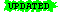CSE 463/563, Spring 2005

# Proper Subformula

 Last Update: 25 February 2005 Note:ormaterial is highlighted

In lecture, I have used the expression "proper subproposition" or sometimes "proper subformula" or even "proper subwff".

Here is a recursive definition of "proper subwff" for our language for FOL.

Let,,be wffs.
Then:

1. Ifis ¬, thenis a proper subwff of.
2. Ifis (v), thenandare proper subwffs of.
3. Ifis (^), thenandare proper subwffs of.
4. Ifis (), thenandare proper subwffs of.
5. Ifis (), thenandare proper subwffs of.

6. Let Q be a quantifier (eitheror).
Let v be a variable.
Then ifis Qv., thenis a proper subwff of.

• Note thatis not necessarily a "sentence" (i.e., a wff with no free variables), since the variable v can occur free in.

7. Ifis a proper subwff of, andis a proper subwff of, thenis a proper subwff of.
8. Nothing else is a proper subwff.

#### An Example:

The proper subwffs of:

• ((¬P¬(Q ^ R)) v ((S ^ T) v (U ^ P)))
are:

• (¬ P¬(Q ^ R))
• ((S ^ T) v (U ^ P))
• ¬P
• ¬(Q ^ R)
• (S ^ T)
• (U ^ P)
• P
• (Q ^ R)
• S
• T
• U
• Q
• R

You can make a nice (recursive) tree diagram with the main wff at the root, and the proper subwffs as children; the leaves will be the atomic wffs. (However, in the case of an FOL wff, some of the leaves might not be sentences, because of the presence of free variables.)